﻿ 重载组合列车跳钩事故原因分析及改善建议

# 重载组合列车跳钩事故原因分析及改善建议Analysis and Improvement Suggestions for the Coupler Separation of Heavy Haul Combined Trains

Abstract: The 20,000 t heavy haul combined train plays the main role of heavy haul transportation. But in the process of train operation, coupler separation and derailment accidents occur from time to time, threatening the security of train operation. A coupler separation accident of a 20,000 t heavy haul train occurred on a heavy-haul line recently. The accident process was reproduced by using the Train Air Brake and Longitudinal Dynamics Simulation System, and the cause of coupler separation of the train after release was analyzed. Improvement suggestions were proposed, providing a reference for the safe operation of heavy haul combined trains.

1. 引言

2. 列车纵向动力学及空气制动系统

2.1. 列车纵向动力学模型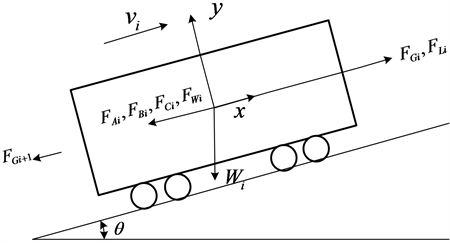Figure 1. The force diagram of a single vehicle

${F}_{1i}={F}_{Gi}-{F}_{Gi+1}+{F}_{L}{}_{i}-{F}_{Wi}$ (1)

${F}_{2i}=\left\{\begin{array}{l}-\frac{{v}_{i}}{|{v}_{i}|}\left({F}_{Ai}+{F}_{Bi}+{F}_{Ci}\right)\text{}{v}_{i}>0\hfill \\ -\text{}\left({F}_{Ai}+{F}_{Bi}+{F}_{Ci}\right)\text{}{v}_{i}=0\text{}and\text{}{F}_{1i}\ge \left({F}_{Ai}+{F}_{Bi}+{F}_{Ci}\right)\hfill \\ \text{}-\text{}{F}_{1i}\text{}{v}_{i}=0\text{}and\text{}{F}_{1i}<\left({F}_{Ai}+{F}_{Bi}+{F}_{Ci}\right)\text{}\hfill \\ \text{}0\text{}{v}_{i}=0\text{}and\text{}{F}_{1i}=0\text{}\hfill \end{array}$ (2)

${m}_{i}{\stackrel{¨}{x}}_{i}={F}_{1}{}_{i}+{F}_{2i}$ (3)

2.2. 列车空气制动系统模型

$\left\{\begin{array}{l}\frac{\partial \rho }{\partial t}+\rho \frac{\partial u}{\partial x}+u\frac{\partial \rho }{\partial x}+\frac{\rho u}{F}\frac{dF}{dx}=0\hfill \\ \frac{\partial u}{\partial t}+u\frac{\partial u}{\partial x}+\frac{1}{\rho }\frac{\partial p}{\partial x}+\frac{4f}{D}\frac{{u}^{2}}{2}\frac{u}{|u|}=0\hfill \\ \frac{\partial p}{\partial t}+u\frac{\partial p}{\partial x}-{a}^{2}\frac{\partial \rho }{\partial t}-{a}^{2}u\frac{\partial \rho }{\partial x}-\left(k-1\right)\rho \left(q+u\frac{4f}{D}\frac{{u}^{2}}{2}\frac{u}{|u|}\right)=0\hfill \end{array}$ (4)

3. 列车跳钩事故重现

3.1. 事故列车运行情况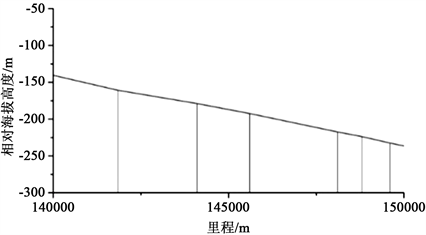Figure 2. The relative altitude map of coupler separation section

3.2. 列车操纵仿真结果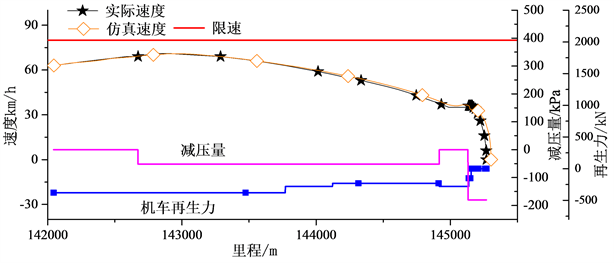Figure 3. The operating instructions and simulate speed of the coupler separation train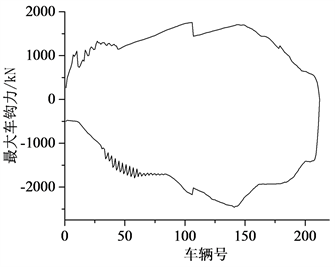Figure 4. The distribution of the maximum coupler force of the train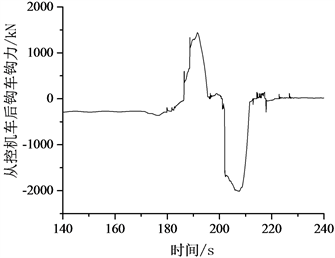Figure 5. The time-field curve of coupler force of slave locomotive’s back coupler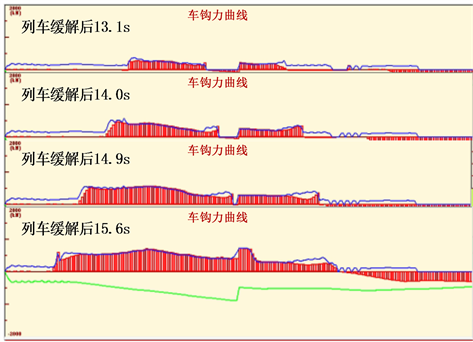Figure 6. The formation process of the first mutant coupler force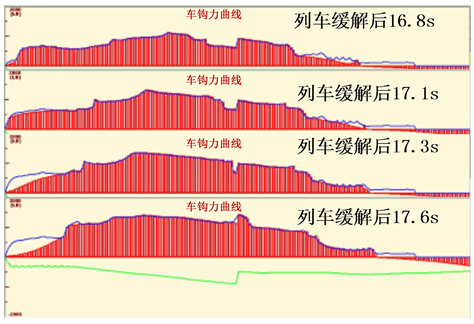Figure 7. The formation process of the second mutant coupler force

4. 操纵改善方案及结果分析

4.1. 方案探究及结果分析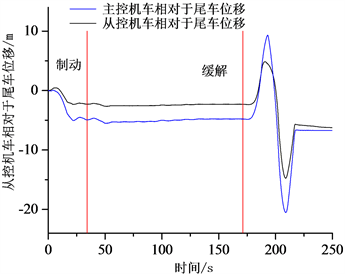Figure 8. The relative displacement of the tripper car and locomotive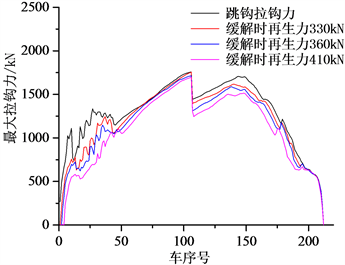Figure 9. The distribution of the maximum coupler force of the train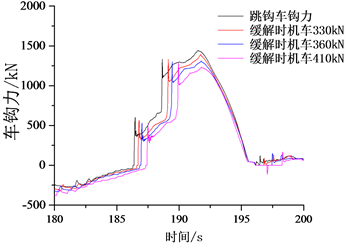Figure 10. The time-field curve of coupler force of slave locomotiveTable 1. The Comparison between the mutant coupler force and the maximum tensile coupler force of the back coupler of the slave locomotive with the original operating instructions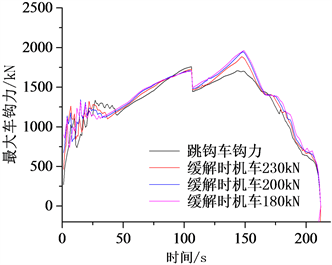Figure 11. The distribution of the maximum coupler force of the train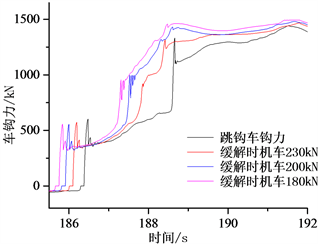Figure 12. The time-field curve of coupler force of slave locomotiveTable 2. The Comparison between the mutant coupler force and the maximum tensile coupler force of the back coupler of the slave locomotive with the original operating instructions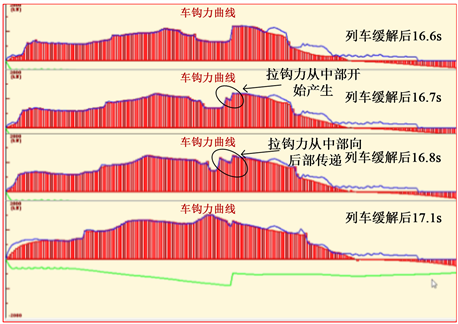Figure 13. The formation process of the tensile coupler force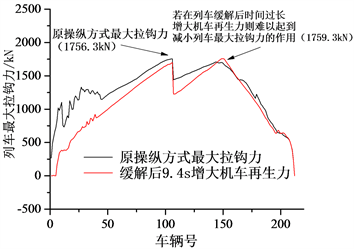Figure 14. The distribution of the maximum coupler force of the train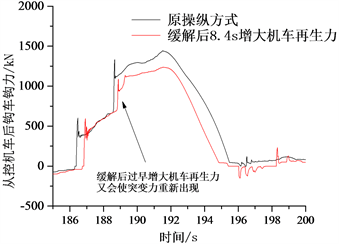Figure 15. The time-field curve of coupler force of slave locomotive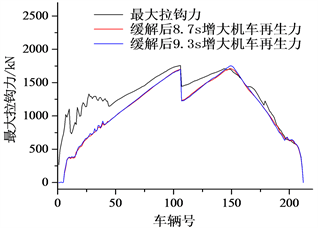Figure 16. The distribution of the maximum coupler force of the train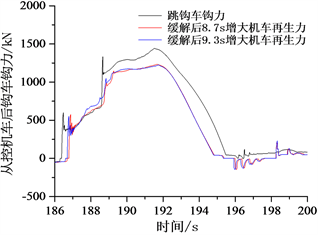Figure 17. The time-field curve of coupler force of slave locomotive

4.2. 避免跳钩的操纵方案汇总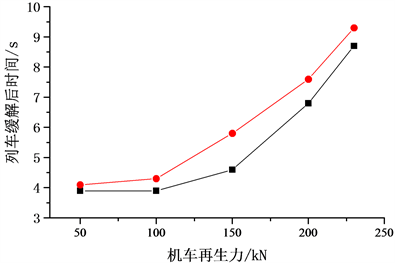Figure 18. The feasible operating instructions of locomotive regenerative braking force

5. 结论

1) 在此次列车的跳钩事故中，列车缓解后在从控机车后钩处产生的两个突变拉钩力是导致列车跳钩的重要原因，这两个突变力由列车缓解后列车后部拉钩力传递至从控机车后钩处产生。

2) 在列车缓解时，通过同步减小机车再生力的操纵方式可使从控机车后钩不会产生第二个突变力，但此操纵方式会使列车缓解后最大拉钩力增大。

3) 在列车缓解时降低机车再生力，配合合适的时间增大机车再生力，可使列车突变拉钩力消失的同时降低列车最大拉钩力，降低列车发生跳钩的可能性。

 Belforte, P., Cheli, F., Diana, G., et al. (2008) Numerical and Experimental Approach for the Evaluation of Severe Longitudinal Dynamics of Heavy Freight Trains. Vehicle System Dynamics, 46, 937-955.
https://doi.org/10.1080/00423110802037180

 Dmitry, P., Vladislav, Y., Nikolay, L., et al. (2017) Train 3D: The Technique for Inclusion of Three-Dimensional Models in Longitudinal Train Dynamics and its Application in Derailment Studies and Train Simulators. Vehicle System Dynamics, 55, 583-600.
https://doi.org/10.1080/00423114.2016.1273532

 魏伟, 张东芹, 张军. 重载列车纵向冲动机理及参数影响[J]. 大连交通大学学报, 2011, 32(1): 1-6.

 耿玮平. 重载列车13A型车钩分离的原因分析和防范建议[J]. 中国高新科技, 2018(16): 112-114.

 伏远昱. 大秦线2.1万吨列车中部机车与车辆车钩分离问题初探[J]. 铁道机车车辆, 2020, 40(4): 109-111+115.

 刘爽, 魏伟. 重载列车安全运行非稳态冲动模型研究[J]. 机械工程学报, 2014, 50(10): 127-142.

 岳刚. 货车提速后车钩分离的原因分析及建议[J]. 机车车辆工艺, 2004(2): 36-38+46.

 丁国斌. 大秦线运营车辆13号车钩区间分离故障的原因分析及防止措施[J]. 铁道机车车辆, 2004(1): 56-59.

 魏伟, 李文辉. 列车空气制动系统数值仿真[J]. 铁道学报, 2003(1): 38-42.

 宋健. 重载列车纵向动力学试验与仿真的比较研究[D]: [硕士学位论文]. 大连: 大连交通大学, 2018.

 魏伟, 赵旭宝, 姜岩, 等. 列车空气制动与纵向动力学集成仿真[J]. 铁道学报, 2012, 34(4): 39-46.

 Wu, Q., Spiryagin, M., Cole, C., et al. (2018) International Benchmarking of Longitudinal Train Dynamics Simulators: Results. Vehicle System Dynamics, 56, 343-365.
https://doi.org/10.1080/00423114.2017.1377840

Top